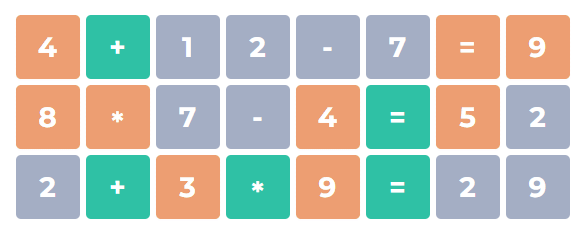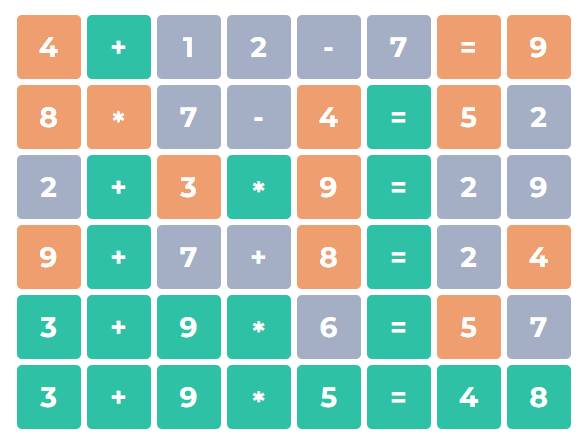# Numberle Game: Guess the Math Equation

## How to play Numberle?

1. ### Enter your own equation

To start the game, simply enter any correct equation to find clues. In total, you will have 6 tries to guess the target equation. When calculating, you can use numbers (0-9) and arithmetic signs (+ - * / =).2. ### Find out what numbers and signs are in the equation

If there are any numbers or arithmetic signs in the target equation, but in the wrong place, it will be highlighted in brown. If there are numbers or signs in the equation and in the exact place, then it will be highlighted in green. Gray color means that these numbers or signs are not in the equation.3. ### Try to solve the target equation

To win the game, you need to guess the equation exactly (all spots are green). At the end of the game, you can easily share your result on social media, as well as copy the link and challenge your friends!## What is Numberle?

Numberle is a math puzzle game inspired by Wordle, the game that made a splash in early 2022. The main goal of the Numberle game is to accurately guess the mathematical equation in 6 tries. As you enter your own equations, you'll see colored hints that indicate how close you are to solving the puzzle, and if all the rows are highlighted in green, then you have won! This game is perfect for training your brain and having fun with your friends.